Residual is the difference between the observed value and the predicted value. Observed value is the actual data point while predicted value is the value obtained from the regression equation. On a residual plot, it is the vertical difference between the observed point and the line

Residual Analysis is the study of residual plots to validate the goodness of a regression model. For a good model, the residuals have to be random and normally distributed

An application-oriented question on the topic along with responses can be seen below. The best answer was provided by Senthilkumar G on 19th Jun 2020

Applause for all the respondents - Raj Saxena, Rajeshwari, Senthilkumar G, Tushar Maradwar

Also review the answer provided by Mr Venugopal R, Benchmark Six Sigma's in-house expert.

## Question

Q 271. What is a residual in Regression? Why is it important to analyze the residuals before assessing the goodness of a Regression Model? What does it mean if Residuals are non normal or non random?

Note for website visitors - Two questions are asked every week on this platform. One on Tuesday and the other on Friday.

## Recommended Posts

• 0

Q 271. What is a residual in Regression? Why is it important to analyze the residuals before assessing the goodness of a Regression Model? What does it mean if Residuals are non normal or non random?

Residual:

Cutting-edge statistics and optimization, Residuals and statistical errors are closely related and easily disordered measures of the deviation of an observed value of an element of a statistical from its “Theoretical Value”.

Residual = Observed value - Predicted value

The Error of an observed value is the deviation of the observed value from the true value of quantity of interest (for example: a population mean) and the residual of an observed value is the variance between the observed value and the estimated value of the quantity of interest (for example:  a sample mean). The division is most important in regression analysis, where the concepts are sometimes called the regression statistical errors and regression residuals and where they lead to the concept of studentized residuals.

Error Vs Residual:

·         The difference between the height of each person in the sample and the unobservable population mean is a statistical error, whereas

·         The difference between the height of each person in the sample and the observable sample mean is a residual.

Residual in Regression:

Since a linear regression model is not always appropriate for the data, you should assess the appropriateness of the respective model by defining residuals and examining residual plots.

Residual (e) is the difference between the observed value of the dependent variable (y) and the predicted value (ŷ) and each data point has one residual.

Residual = Observed value - Predicted value
e = y - ŷ

Together the sum and the mean of the residuals are equal to zero ( Σ e = 0 and e = 0).

Notation:

e = Residual

y = Observed Value

y’ = Predicted Value

Properties:

Σ e = 0

Mean of the residuals e = 0

Important to analyze the Residual Plots:

A residual plot is a chart that shows the residuals on the vertical axis and the independent variable on the horizontal axis. The facts in a residual plot are randomly dispersed around the horizontal axis, then linear regression model is mostly appropriate for the particular data set; otherwise, a nonlinear model is more appropriate.

The below table shows the inputs and outputs from a simple linear regression analysis.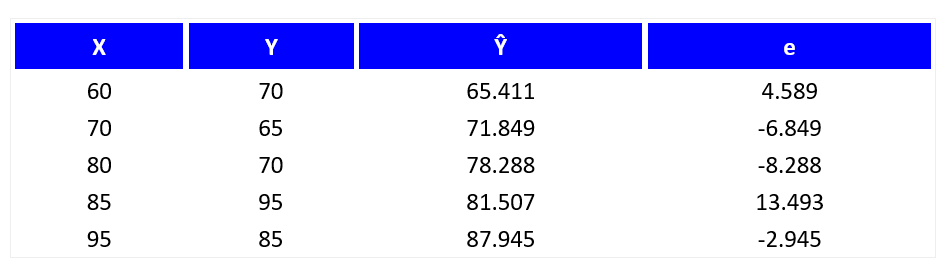The below chart displays the residual (e) and independent variable (X) as a residual plot.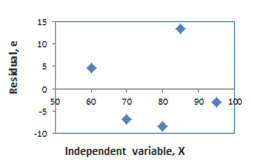The above residual plot shows a fairly random pattern, the first residual is positive and then the next two residuals are negative, the fourth one is positive residual, and the last residual is negative. This random pattern is clearly indicating that a linear model provides a moderate fit to the data.

Below, the residual plots show three typical patterns for the reference. The following first plot shows a random pattern, indicating a good fit for a linear model.

1)     Random Pattern: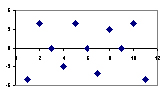2)     Non-Random: U – Shaped: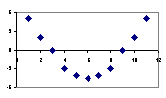3)     Non-Random: Inverted U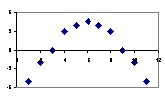The above last two patterns are non-random (U-shaped and inverted U), suggesting a better fit for a nonlinear model.

Residuals are non normal or non random:

Non-normality or non-random of the residual plot is an indication of an inadequate model. It means that the errors the model makes are not consistent cross-ways variables and observations (ie. the errors are not random).

Transformations of Variables:

Once a residual plot data set to be nonlinear, it is commonly possible to "transform" the raw data to make it more linear and it will allow us to use linear regression techniques more effectively with nonlinear data.

What is a Transformation to Achieve Linearity?

Converting a variable involves using a mathematical operation to change its measurement scale. Generally, there are two kinds of transformations.

i)                   Linear transformation. A linear transformation preserves linear relationships between variables. Therefore, the correlation x and y would be unchanged after a linear transformation.

ii)                 Nonlinear Transformation:  Nonlinear transformation changes (increases or decreases) linear relationships between variables and, therefore, changes the correlation between variables.

By using Regression, a transformation to achieve linearity is a special kind of nonlinear transformation. The respective nonlinear transformation that increases the linear relationship between two variables.

Methods of Transforming Variables to Achieve Linearity:

There are numerous ways to transform variables to achieve linearity for regression analysis. Some common methods are summarized below.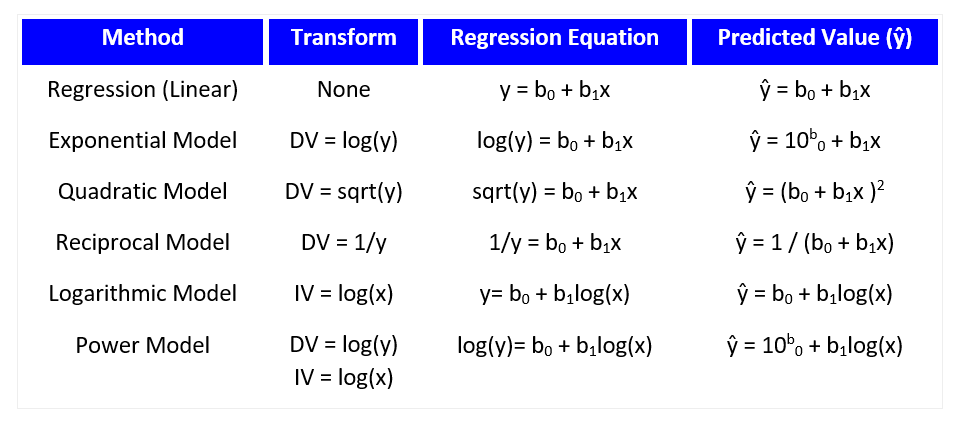Perform a Transformation to Achieve Linearity:

Changing a data set to enhance linearity is a multi-step, trial-and-error process method.

The following steps to be performed for Transforming a data set to enhance Linearity:

i)                   Conduct a standard regression analysis on the raw data.

ii)                  Construct a residual plot.

a.     The plot pattern is random, then do not transform data.

b.     The plot pattern is not random, then continue.

iii)               Compute the coefficient of determination (R2).

iv)               Choose a transformation method (see above table).

v)                Transform the independent variable, dependent variable, or both.

vi)               Conduct a regression analysis, using the transformed variables.

vii)              Compute the coefficient of determination (R2), based on the transformed variables.

a. The transformed R2 is greater than the raw-score R2, then the transformation was successful.

b. If not, attempt a different transformation method.

The greatest transformation method (exponential model, quadratic model, reciprocal model and etc.) will depend on nature of the original data. Healthier way to determine which method is best is to try each and compare the result (residual plots, correlation coefficients). The finest method will yield the highest coefficient of determination (R2).

Reference:

Thanks and Regards,

Senthilkumar Ganesan,

Email: senthillak@gmail.com

Mobile: +91-7598124052.##### Share on other sites
• 1

Benchmark Six Sigma Expert View by Venugopal R

Regression shows us the relationship between two variables, graphically as well as by an equation. By fitting a regression model between two variables, we can predict the dependent variable for any given value of the independent variable.

What is residual?

One of the commons methods used to fit a regression line is known as 'The Least Square Residual' method. On a fitted regression, many of the observed values would not fall exactly on the fitted line. The vertical distance between the observed line and the fitted line is the residual value. The residual can be either positive or negative depending on whether the observed value falls above the fitted line or below.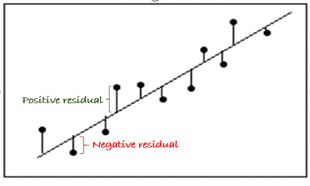Residual and Goodness of Fit

The residuals and their pattern represent how the errors are distributed along the regression fit. By assessing the pattern of the residuals, we can determine whether they represent a stochastic error pattern. By stochastic, we mean whether the error pattern is random and unpredictable.

For a simple analogy, we may take the case of a 'Rolling of dice' example. Though we would not be able to predict the outcome when an unbiased dice is rolled each time, over a series of tosses, we can determine whether the appearing numbers follow a random pattern or not. For instance,  with a biased dice, if we observe the number “3” appearing more number of times, there is a bias and if this is noticed by a player, can be used to his / her advantage.

Similar principle applies to regression models as well. For a series of observations, the errors should be random and unpredictable. By analyzing the residuals, we will be able to decide whether the regression model represents a systematically correct and reliable model or whether we need to improve it.

Randomly distributed residuals

For simplicity, we will stick our discussion to Ordinary Least Square regression model (OLS). The plot below is that of the residuals, plotted by taking zero as the X axis. We get this plot by choosing Residual vs Fits option in Minitab.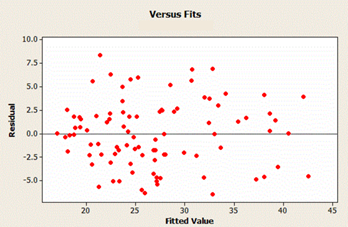Since natural variations tend to follow normal distributions with more values falling close to the center and symmetrically spread on both sides, the residuals are also expected to show randomness and spread across horizontally as shown above to represent a systematic OLS model. The similar pattern of spread across the breadth shows the level of randomness is maintained across the range of the relationship. This behavior is also known as homoscedasticity or constant variance.

Non randomly distributed residuals

The below picture depicts a possibility of heteroscedasticity, or non-constant variance.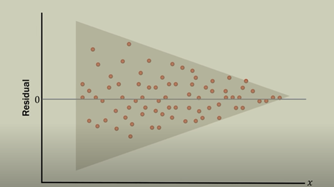Here, the variation of the residuals is higher towards the left and it reduces as we go to the right. This pattern represents that the error is higher during the regression relationship for the lower set of values and decreases significantly as we move to the higher sets of values. Heteroscedasticity does not cause bias on the estimation of coefficients, but it adversely affects their precision. This pattern of variation violates the assumptions of the Linear Regression Modelling and becomes unreliable for predictions.

Non normally distributed residuals

Another type of Heteroscedasticity is shown below.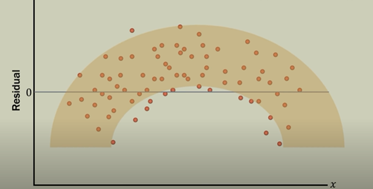In this case, it is a nonlinear data and hence the wrong model. The residuals follow an arch like shape. This indicates that the data is nonlinear and applying linear model is a mistake. In this example, the residuals will be non-normal and skewed to one side.

What do heteroscedastic models indicate, in general?

The heteroscedastic models indicate the some deterministic component of the model, i.e. predictor variable is not capturing some assignable or explanatory information and allowing it to get added into the residuals.

Or it could be an interaction between the variables across the levels, which had not been identified. For example, if we want to study the productivity of workers across age, we might have a situation where the variation of the performance could be less for the younger ages and as the age increases there could be drop in productivity, coupled with an increased variation. This will result in a conical shaped distribution with increasing variation along the X axis of the ‘Residual vs Fits’ graph.

##### Share on other sites
• 0

What is a residual in Regression?

A Residual is a Perpendicular distance between a data point and the regression line.

A Residual is a measure of how well a line fits and individual point.

Each data point has one residual. They are positive if they are above the regression line and negative if they are below the regression line. And if the regression line actually passes through the point, the residual at that point is Zero.

You get a line of best fit while performing Simple line regression and the data point don’t fall exactly on this regression equation line, but scattered around it.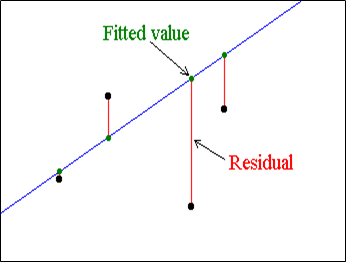Why is it important to analyze the residuals before assessing the goodness of a Regression Model?

It should assess the goodness of the model by defining residuals and examining residual plot because a linear regression model is not always appropriate for the data.

-          If we violate the assumptions, we risk producing results that can’t be trusted.

-          If plots display unwanted patterns then can’t trust regression coefficients and other numeric results.

-          If you do see unwanted pattern in residual plot, it actually represents a chance to improve your model because there is something more that your independent variables can explain.

-          It generates unbiased Coefficient estimates that tend to be relatively close to the true population values (minimizing the variance).

-          It helps you determine whether a linear model is adequate for your data.

What does it mean if Residuals are non normal or non random?

Non Normal

This means the hypothesis that they are a random dataset, takes the value No. This means that regression model doesn’t explain all trends and model is not fully explaining the behavior of system.

Also in the error in model is not consistent across the full range of observed data. And the amount of predictive ability the have is not the same across the full range of the dependent variable. Hence predictors technically mean different things at different levels of dependent variable.

Non Random

The Non Random pattern in the residuals indicates that the deterministic portion (Predictor variable) of the model is not capturing some explanatory information that is leaking into the residuals.

What could be the possibilities includes in Non Random in residuals.

1.       Missing Variable

2.       Missing  interaction between terms in your existing model

3.       Missing higher-order variable terms that explain a non-linear pattern.

Thanks to resource:-

##### Share on other sites
• 0

Residual is a measure of error and tells us the variance or how far is the predicted value from the actual value. If our predicted value is greater than the actual value the residual is negative. If predicted value is smaller than the actual value the residual is positive. The size of the residual would explain how fa we are from the actual value.

Basically we create a scatter plot based on the sample data and draw the line of best fit that would approximate the trend and be the line that would be closest to all the points, that would be the regression line which can be represented as y=mx+b

residual is good for saying how good the line is , does the regression  and the model fit a given data point.

When we look at the combination of all the residuals and try to minimize it, adding all of them would not be the ideal approach since we could have both positives and negatives netting off and in turn showing the value either as zero or minimal which does not reflect the right picture instead we could add the sum of all the residuals as absolute number. Residuals are also called errors since it is the error that is not explained by the regression line.

It is important  to analyze the residuals before assessing the goodness of a regression model since it would tell us how accurate the model is that we are building.Also Linear regression is not always appropriate for the data, hence we need to assess the appropriateness of the model by evaluating the residual plots.

Non Normal residuals means variance or inconsistency across the variables and observations and we calculate prediction intervals in a model assuming that the residuals or normal,however if the data is non normal the predictions might not be accurate  in such cases we need to look at data and check the distribution and understand if there are any special causes contributing for the same.

##### Share on other sites
• 0

What is a residual in Regression?

In Regression analysis we get a line of best fit which is know as “Regression equation line” the data points usually get scattered around  this regression equation line. A residual is the vertical distance between a data point and the regression line. Refer to below fig. 1, the length of the red line segments (D1,D2,D3,D4,D5) are called RESIDULES.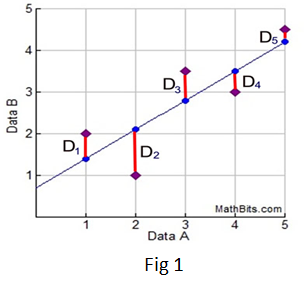Mathematically also we can understand it with below formula

Residual = (Observed y value on scatter plot - Predicted yvalue on Regression equation line)

Refer to above formula and figure we can calculate Residual as per below

D1 = (2 - 1.4) = 0.6
D
2 = (1 - 2.1) = -1.1
D
= (3.5 - 2.8) = 0.7
D
4 = (3 - 3.5 )= -0.5
D
5 = (4.5 - 4.2) = 0.3

• If we add all values from D1 to D5 its summation is zero (0.6-1.1+0.7-0.5+0.3=0) , it means the sum of the residuals always equals zero.
• Similarly the mean of residuals is also equal to zero, as the mean = the sum of the residuals / the number of items.
• In Regression, each data point has one residual ,if they are above the regression line they are positive (e.g D1,D3 & D5 in above figure) and negative if they are below the regression line (e.g D2 & D4 in above figure) . If the regression line actually passes through the point, the residual at that point is zero.

Why is it important to analyze the residuals before assessing the goodness of a Regression Model?

• By analyzing Residuals it help to determine the validity of model, and give information about model whether it is making any systematic error or not,
• By validating Residuals plots , we come to know whether model is biased or not, if model is biased than we cannot trust the results and If residual plots look good, than we can assess R-squared and other statistics.
• By validating Residuals we come to know about randomness and unpredictability of Regression model , if we do not have these two than we can say model is not valid.
• Analysis of Residuals can be done by evaluating Residuals plots, which helps to expose a biased model far more effectively than the numeric output by displaying problematic patterns in the residuals.

A residual plot is used to find problems with regression. Following data sets are not good for regression

• Data that is non-linearly associated.
• Heteroscedastic data
• Data sets with outliers.

For example refer to fig 2 if curvature is present in the residuals, then it is likely that there is curvature in the relationship between the response and the predictor that is not explained by model.  A linear model does not adequately describe the relationship between the predictor and the response. In this example, the linear model systematically over-predicts some values i.e. the residuals are negative , and under predict others i.e. the residuals are positive.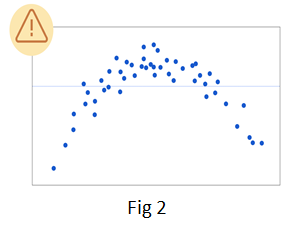Heteroscedasticity-Refer to fig 3, heteroscedasticity happens If the residuals fan out as the predicted values increase, it means that the variability in the response is changing as the predicted value increases.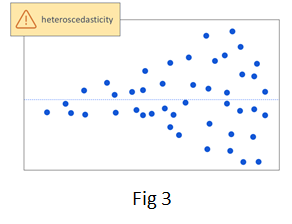Outliers- Outliers can have a big influence on the fit of the regression line. An unusual pattern can also be caused by an outlier. Refer to fig 4, we have one obvious outlier, which is tilting the regression line. As a result, the model will not predict well for many of the observations.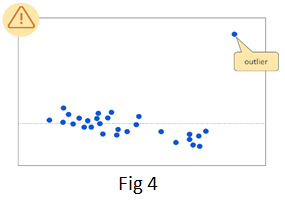What does it mean if Residuals are non normal or non random?

• The meaning of non normality of the Residuals is an indication of an inadequate model, which means that the errors the model makes are not consistent across variables and observations.
• Non-random patterns in residuals indicates that variables are missing something.
• The non-random pattern in the residuals indicates that the predictor variables of the model is not capturing some explanatory information that is leaking into the residuals. The graph could represent several ways in which the model is not explaining all that is possible.

##### Share on other sites
• 0

Senthilkumar has provided the best answer to this question. He has explained residuals, why we need to analyze the residual plots, the meaning of non normal and non random residuals and also highlighted how we could address these issues in regression. Raj's answer is also a must read.

Also review the answer provided by Mr Venugopal R, Benchmark Six Sigma's in-house expert.

##### Share on other sitesThis topic is now closed to further replies.

• ### Who's Online (See full list)

There are no registered users currently online

• ### Forum Statistics

• Total Topics
2,842
• Total Posts
14,364
• ### Member Statistics

• Total Members
54,953
• Most Online
888

×
×
• Create New...